# CNN-卷积神经网络

CNN 的核心思想：局部感受野（local field） + 权值共享 + 亚采样 。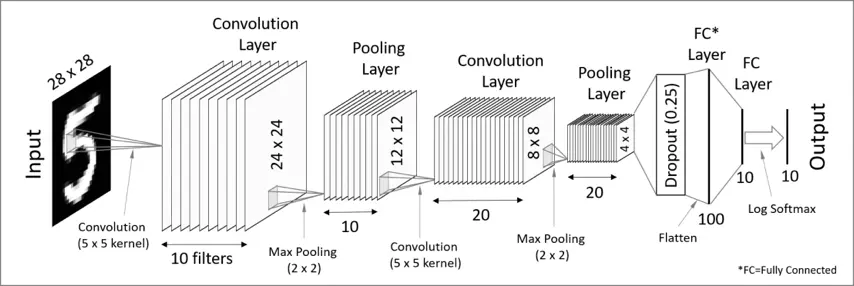CNN 主要由四类层（layer）组成，即卷积层(Conv)、激活层(Activation)、池化层(Pooling) 以及全连接层(FC/Dense)。

## 卷积层（Convolution）

### 卷积层如何生成

##### 卷积核 (或称滤波器，filter/kernel)##### 卷积核的 stride 值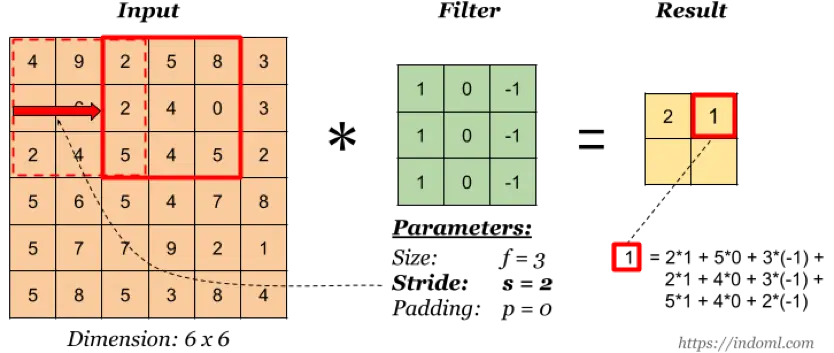stride 指卷积核的移动步长(这其实就是权值共享的表现)，上图 stride=2，卷积核每次移动两个单元。

##### 卷积核的 padding 值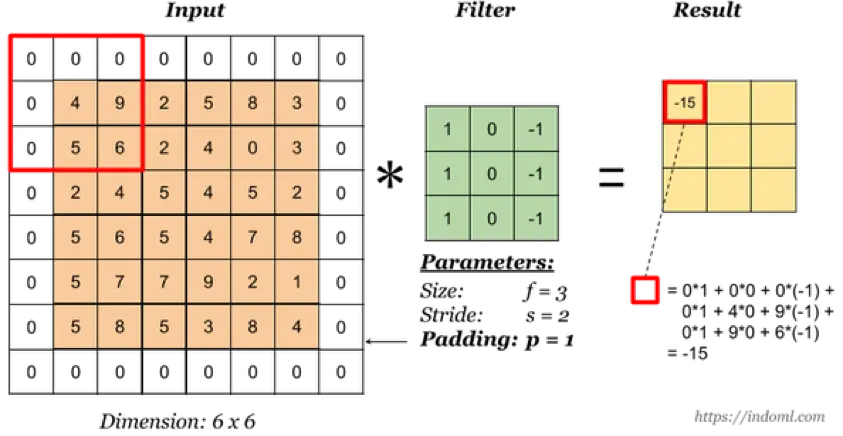padding 即在输入矩阵的四周添加填充，一方面可以减少原输入的边缘影响，另一方面可以用于维持输入和输出的维度一致（需要 stride 配合）。

(1) valid: 即 no padding
(2) same：通过填充使得输入和输出的维度保持一致

##### 多通道卷积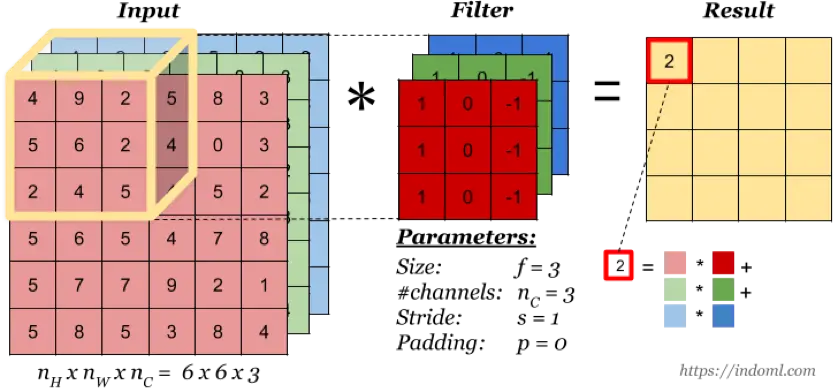##### 多卷积核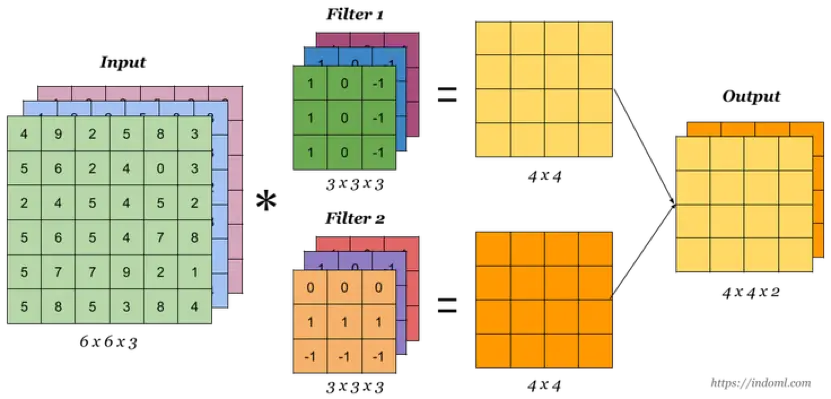# RNN/LSTM-递归神经网络

### 一、RNN vs LSTM

#### RNN 逻辑结构图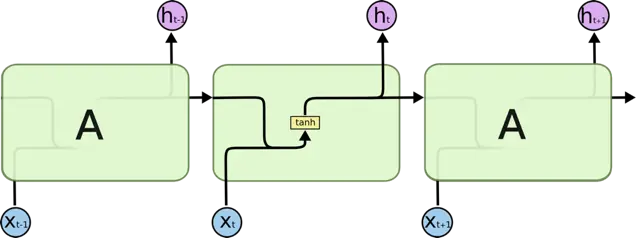#### LSTM 逻辑结构图LSTM 是 RNN 的变体，它们的原理几乎一样，唯一的不同是 output 即 hidden state 的计算逻辑不同

#### RNN 如何计算某个时刻 t 的 output ?

$h_t = tanh(W*[h_{t-1}, x_t]+b)$

#### LSTM 如何计算某个时刻 t 的 output ?

$f_t = sigmoid(W_f*[h_{t-1}, x_t] + b_f)$

${C}^{*} = tanh(W_c*[h_{t-1}, x_t]+b_c)$

$i_t = sigmoid(W_i*[h_{t-1}, x_t] + b_i)$

$C_t = f_t*C_{t-1} + i_t*{C}^{*}$

$o_t = sigmoid(W_o*[h_{t-1}, x_t] + b_o)$

$h_t = o_t*tanh(C_t)$

#### LSTM 为什么优于 RNN ?

RNN 通过叠乘的方式进行状态更新，当 sequence 比较长时容易出现梯度消失/爆炸的情况，主要原因是反向传播的连乘效应，而 LSTM 是通过门控制的叠加方式来更新状态（C_t的计算公式），所以可以有效防止梯度问题，当然对于超长的 sequence，LSTM 依然会有梯度消失或者爆炸的可能。

### 二、RNN/LSTM 中的 num_units 是啥意思 ？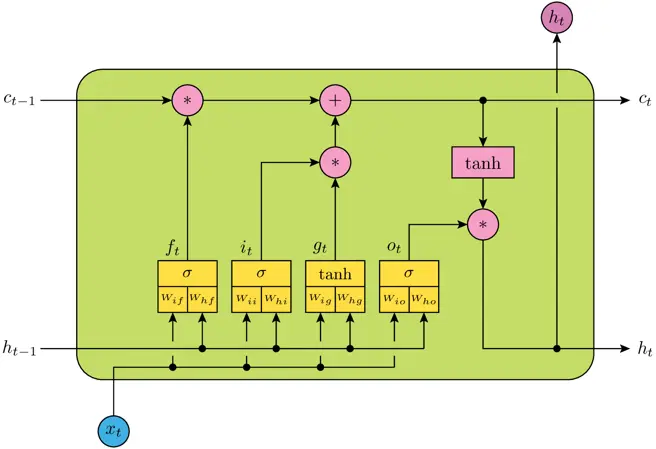num_units 相当于神经网络的隐层神经元的个数，例如上图表示一个 LSTM Cell，包含四个神经网络层，即黄色方框部分，num_units 就是每个神经网络结构的隐层神经元个数(全连接单元数)，它实际上也是 LSTM 输出向量的维度数，所以 h_t 为 num_units 维向量。

### 三、如何计算 Keras 的 LSTM layer 的参数个数？

$(num\_units + input\_dims + 1) * num\_units * 4 = 150600$

1. num_units + input_dims 是因为上层输出需要首先与输入进行一次concat，即 [h_t-1, x_t],
2. + 1 是因为 bias
3. * 4 是因为共有4个神经网络层（黄色方框）
4. 为什么不需要乘以 time_steps 即 number of cell ?

### 五、为什么 LSTM 的输入和输出值不用 sigmoid 而用 tanh 作为激活函数 ？

LSTM 内部维护了一个状态向量，其值应该可以增加或者减少，而 sigmoid 的输出为非负数，所以状态信息只能增加，显然不合适，相反，tanh 的输出范围包含了正负数，因此可以满足状态的增减。

# 基于 CCO 的协同过滤推荐

## 基于 CCO 的协同过滤推荐

#### 基于单一行为

u1=> [ t1, t2, t3, t5 ]
u2=> [ t1, t3, t4, t5 ]
u3=> [ t2, t4 ]# 常用推荐算法比较

## 基于内容推荐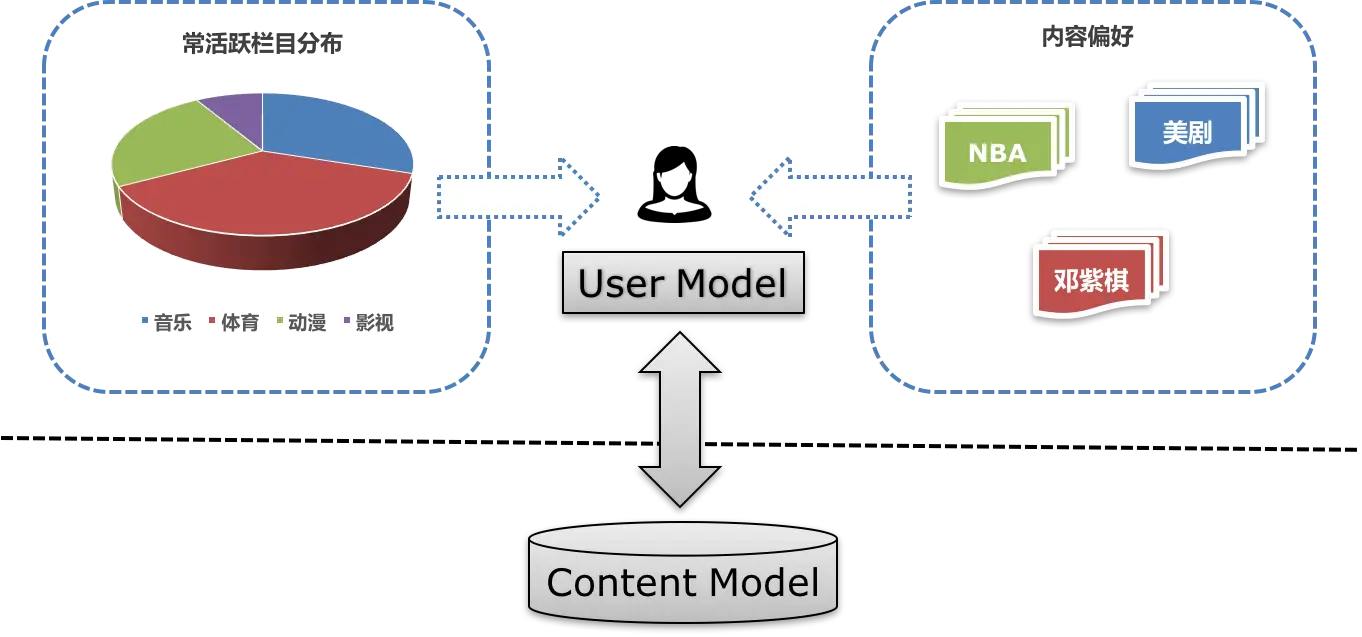## 协同过滤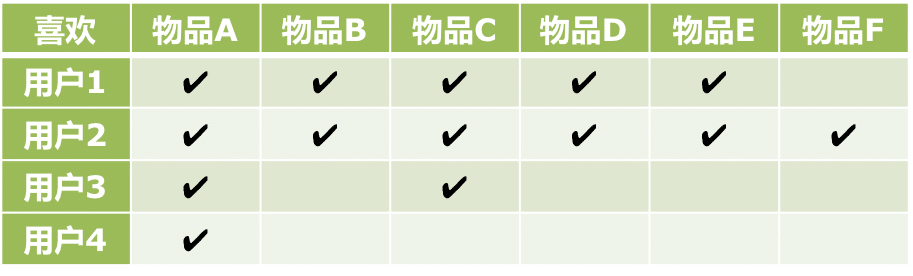# 个性化推荐系统的基本抽象

## 关于推荐

#### 人工 VS 个性化

• 早期的推荐功能大多以人工筛选为主。人工筛选可以确保内容的高质量，这是主要的优点之一，但人工筛选往往需要投入大量的人力成本。另外，由于不同用户的个人偏好差异巨大，高质量的内容往往不等于最合适的内容（例如：一篇介绍奢侈品牌化妆品的“高大上”内容对于一位平时只关心美食和户外运动的用户而言可能是毫无吸引力的）。

• 为了提升用户体验，后来出现了“个性化内容推荐”的概念，通过引入个性化推荐系统，解决这类“千人千面”的问题。

## 推荐系统架构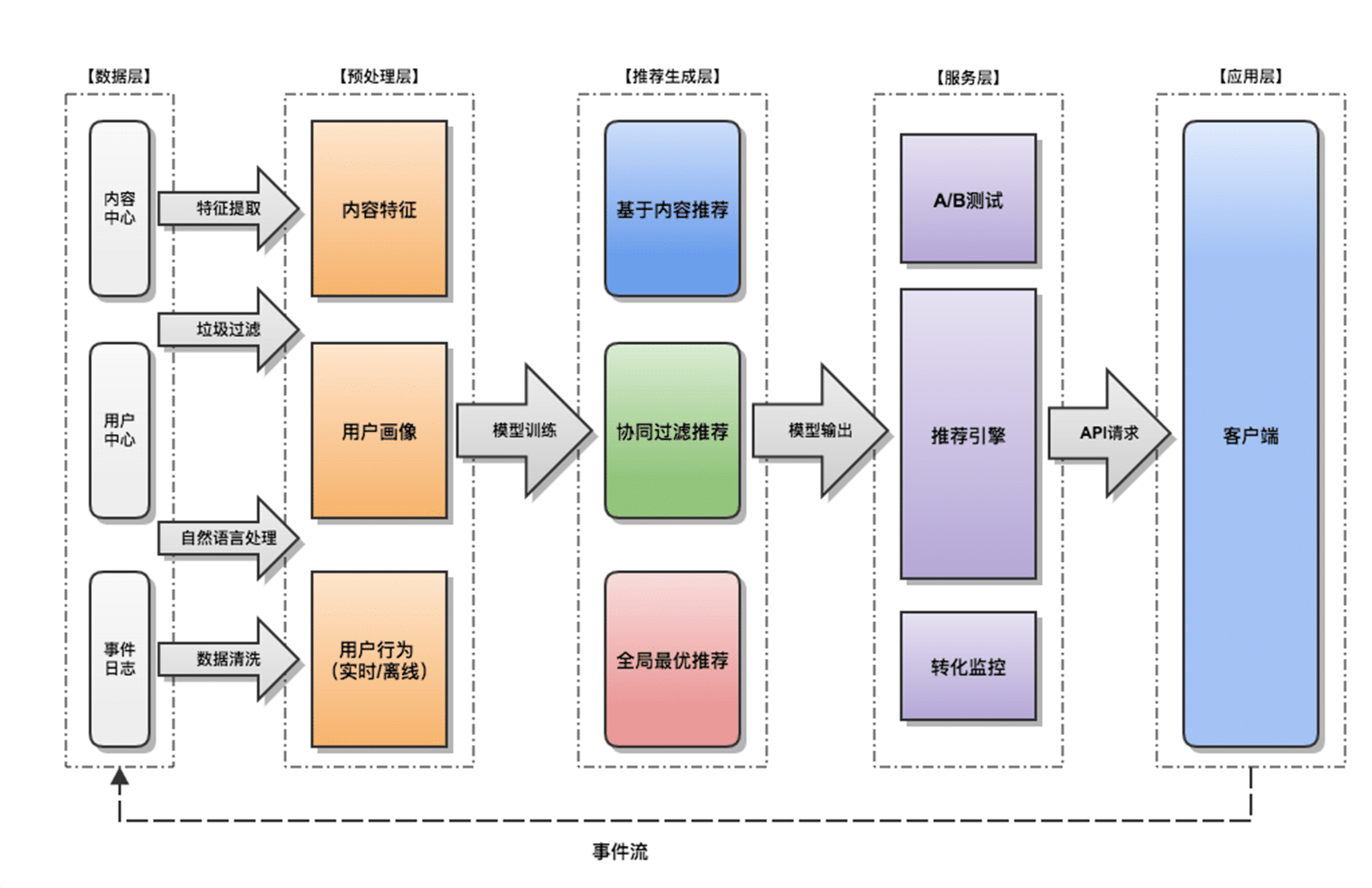# 基于词向量的文本分类推断

## 提取文本标签特征

2016/17赛季欧冠决赛在威尔士卡迪夫千年球场打响，最终尤文图斯以1-4不敌皇家马德里，遗憾错失冠军。赛后，尤文门将布冯表示对结果非常失望，因为尤文已经做了所有能做的事情。

#欧冠#决赛#尤文图斯#皇家马德里#布冯#门将#球场#冠军

• 一级分类：体育
• 二级分类：篮球（关联标签：NBA，CBA，篮球，篮板球，助攻，盖帽，FIBA，姚明，乔丹，三双…）
• 二级分类：足球（关联标签：世界杯，亚冠，欧冠，中超，足球，英超，西甲，梅西，里皮，马拉多纳，门将，广州恒大，曼联…）

## 分类推断

Similarity(tagA, tagB) = cos(tagA_Vec, tagB_Vec)

Dist(doc_tags, soccer_tags) > Dist(doc_tags, basketball_tags)

# 基于 TWE 模型的关键词提取

## TWE (Topical Word Embedding)

#### 主题模型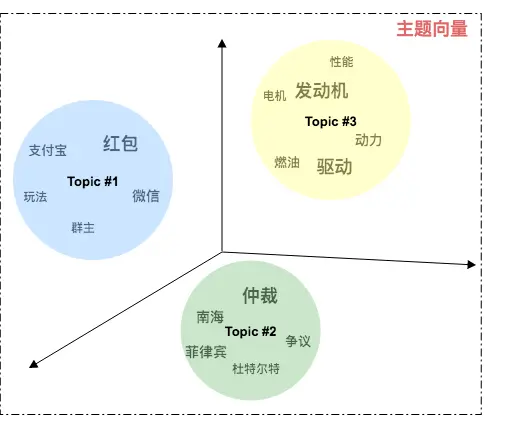• 在主题模型中每个主题实际上是一系列代表该主题的词的分布 ，例如：上图主题 Topic#1 包含的主题词分布主要有 “红包”、“微信”、“支付宝”、“玩法”等，文字大小代表该词的概率分布差异，可以推断 Topic#1 是一个关于新年红包活动的主题。

#### 词向量模型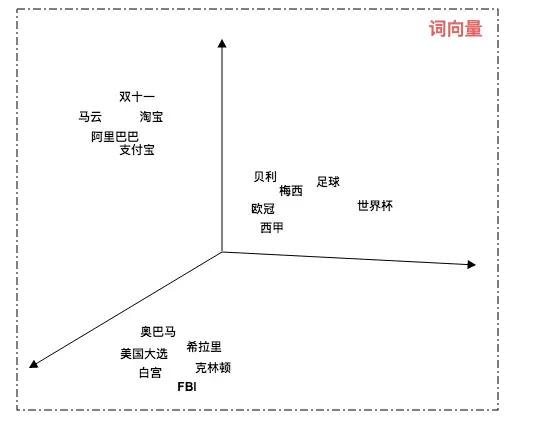• 在词向量模型中每个词都有自己的坐标，关联度越高的词相互的距离越近。例如：“梅西” 和 “足球” 的距离比 “奥巴马” 与 “足球” 的距离要近得多，所以 “梅西” 与 “足球” 的关联度相比 “奥巴马” 更高。

#### 基于 TWE 提取关键词

1. 通过主题模型我们可以知道一段文本的主题分布：P(topic | doc)
2. 结合词向量和主题向量，我们可以通过余弦函数计算两者的距离：cos(word_vec, topic_vec)
3. 通过计算词向量与该文本所有关联主题的主题向量的余弦相似度，最终得到词与文本的语义关联度：
$Sim\displaystyle\text{(word, doc)}=\sum_{k=1}^K \cos(\text{word\_vec, topic\_vec}_{_k}) \times P(\text{topic}_{_k} \mid \text{doc})$


## 算法比较#### 效果评价

1. TF-IDF 更倾向于高频词。
2. TextRank 综合考虑文本结构和词频，但它致命的问题在于，头尾的信息由于只有单边的入度，容易被抛弃掉，例如本例中的核心词 “pagerank”。
3. TWE 更强调语义相关性，实际的提取效果也优于前面两种方式。

# NLP 关键词提取算法之 TextRank

## TextRank 介绍

TextRank 是一种基于图的排序算法，它的基本思想来自于著名的 Google PageRank.#### PageRank 的基本思想

• 一个网页的入链越多，它就越重要。
• 如果一个网页被越重要的网页所指向，它也越重要。

## TextRank 算法简释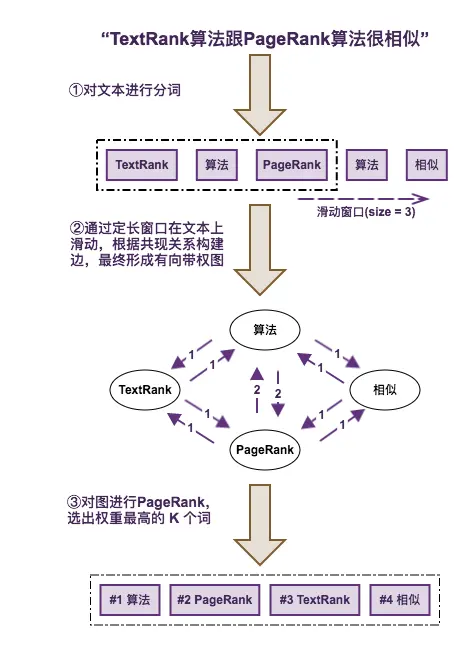#### 通过 TextRank 进行关键词提取的主要步骤

1. 对文本进行分词，并去掉停用词以及非目标词性词汇。
2. 通过一个固定长度的滑动窗口在分词文本上滑动，每个窗口内的词根据共现关系构建一条边，最终形成一个图。
3. 对图进行 PageRank，实际上就是投票和迭代的过程。
4. 选出权重最高的 TopK 个词。

## 算法总结

• TextRank 算法一定程度上考虑了文本中词与词之间的关系，也就是文本结构，在实际应用中它的效果一般会比 TF-IDF 好，当然它的计算复杂度也比 TF-IDF 高得多。
• TextRank 算法更擅长处理长文本，对短文本的效果并不理想，主要因为短文本的词汇信息较弱，构建的图并不理想。
• TextRank 算法仍然倾向于选择出现较为频繁的词作为关键词。

# NLP 关键词提取算法之 TF-IDF

TF-IDF 是处理 NLP 问题时经常使用的经典算法，常用于对文本进行关键词的提取。

## TF-IDF 介绍

#### 计算公式

TF-IDF 的计算非常简单

$TF-IDF = TF(\text{文章词频}) * IDF(\text{逆文档频率})$


#### 概念解释

TF: 指某个词在一段文本中出现的词频

$TF = \dfrac{\text{某个词在文章中出现的次数}}{\text{文章总词数}}$


IDF: 指某个词在所有文本（对应语境所在的训练语料，例如：科学领域、体育领域）中的区分度，它认为越少出现的词区分度越高，相应的 IDF 值也越高。

$IDF = \log\dfrac{(\text{训练语料的总文档数+1})}{(\text{出现该词的文档数+1})}$


## 算法总结

#### 评价

• TF-IDF 计算复杂度低，适合对效率优先的场景。
• 由于它只依赖关键词的统计特征进行排序，有时候效果并不理想，比如有时候文本的关键要素不一定是频繁出现的元素。
• 另外它也缺乏对文本结构的考虑。

# 方差、标准差、均方误差、协方差的区别

#### 方差（Variance）

$σ^2 = \dfrac{(x_1 - \bar{X})^2 + (x_2 - \bar{X})^2 ... + (x_n - \bar{X})^2}{(n-1)} = \dfrac{\sum_{i=1}^n{(x_i - \bar{X})^2}}{(n-1)}$



#### 标准差（Standard Deviation）

标准差 = 方差的算术平方根

$σ = \sqrt{\dfrac{(x_1 - \bar{X})^2 + (x_2 - \bar{X})^2 ... + (x_n - \bar{X})^2}{(n-1)}} =\sqrt{\dfrac{\sum_{i=1}^n{(x_i - \bar{X})^2}}{(n-1)}}$


#### 均方误差（Mean Squared Error）

y:预测值 ， Y:真实值

$MSE = \dfrac{(y_1 - Y_1)^2 + (y_2 - Y_2)^2 ... + (y_n - Y_n)^2}{n} = \dfrac{\sum_{i=1}^n{(y_i - Y_i)^2}}{n}$


#### 协方差 (Covariance)

$Cov(x,y) = \dfrac{(x_1 - \bar{X})(y_1 - \bar{Y}) + (x_2 - \bar{X})(y_2 - \bar{Y}) ... + (x_n - \bar{X})(y_n - \bar{Y})}{(n-1)} = \dfrac{\sum_{i=1}^n{(x_i - \bar{X})(y_i - \bar{Y})}}{(n-1)}$